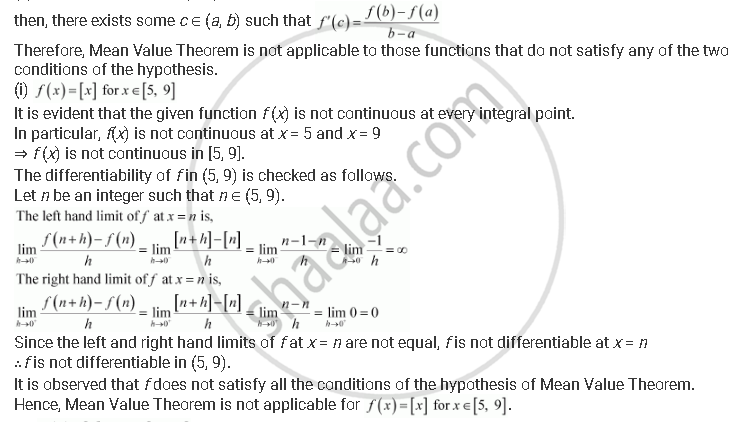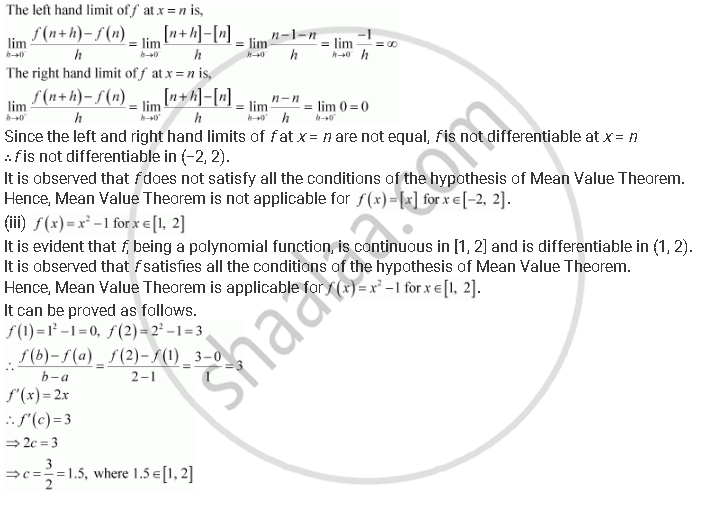Share

# Examine the Applicability of Mean Value Theorem for All Three Functions Given in the Above Exercise 2. - CBSE (Science) Class 12 - Mathematics

#### Question

Examine the applicability of Mean Value Theorem for all three functions given in the above exercise 2.

#### Solution

Mean Value Theorem states that for a function f:[a,b] -> R, if

(a) f is continuous on [ab]

(b) f is differentiable on (ab)ii) f (x) = [x] for x ∈ [– 2, 2]

It is evident that the given function f (x) is not continuous at every integral point.

In particular, f(x) is not continuous at = −2 and = 2

⇒ f (x) is not continuous in [−2, 2].

The differentiability of f in (−2, 2) is checked as follows.

Let be an integer such that n ∈ (−2, 2).Is there an error in this question or solution?

#### APPEARS IN

NCERT Solution for Mathematics Textbook for Class 12 (2018 to Current)
Chapter 5: Continuity and Differentiability
Q: 6 | Page no. 186

#### Video TutorialsVIEW ALL 

Solution Examine the Applicability of Mean Value Theorem for All Three Functions Given in the Above Exercise 2. Concept: Mean Value Theorem.
S